# matplotlib.pyplot.figure¶

matplotlib.pyplot.figure(num=None, figsize=None, dpi=None, facecolor=None, edgecolor=None, frameon=True, FigureClass=<class 'matplotlib.figure.Figure'>, clear=False, **kwargs)[source]

Create a new figure, or activate an existing figure.

Parameters
numint or str or Figure, optional

A unique identifier for the figure.

If a figure with that identifier already exists, this figure is made active and returned. An integer refers to the Figure.number attribute, a string refers to the figure label.

If there is no figure with the identifier or num is not given, a new figure is created, made active and returned. If num is an int, it will be used for the Figure.number attribute, otherwise, an auto-generated integer value is used (starting at 1 and incremented for each new figure). If num is a string, the figure label and the window title is set to this value.

figsize(float, float), default: rcParams["figure.figsize"] (default: [6.4, 4.8])

Width, height in inches.

dpifloat, default: rcParams["figure.dpi"] (default: 100.0)

The resolution of the figure in dots-per-inch.

facecolorcolor, default: rcParams["figure.facecolor"] (default: 'white')

The background color.

edgecolorcolor, default: rcParams["figure.edgecolor"] (default: 'white')

The border color.

frameonbool, default: True

If False, suppress drawing the figure frame.

FigureClasssubclass of Figure

Optionally use a custom Figure instance.

clearbool, default: False

If True and the figure already exists, then it is cleared.

tight_layoutbool or dict, default: rcParams["figure.autolayout"] (default: False)

If False use subplotpars. If True adjust subplot parameters using tight_layout with default padding. When providing a dict containing the keys pad, w_pad, h_pad, and rect, the default tight_layout paddings will be overridden.

constrained_layoutbool, default: rcParams["figure.constrained_layout.use"] (default: False)

If True use constrained layout to adjust positioning of plot elements. Like tight_layout, but designed to be more flexible. See Constrained Layout Guide for examples. (Note: does not work with add_subplot or subplot2grid.)

**kwargs : optional

See Figure for other possible arguments.

Returns
Figure

The Figure instance returned will also be passed to new_figure_manager in the backends, which allows to hook custom Figure classes into the pyplot interface. Additional kwargs will be passed to the Figure init function.

Notes

If you are creating many figures, make sure you explicitly call pyplot.close on the figures you are not using, because this will enable pyplot to properly clean up the memory.

rcParams defines the default values, which can be modified in the matplotlibrc file.

## Examples using matplotlib.pyplot.figure¶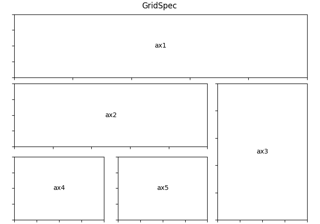Using Gridspec to make multi-column/row subplot layouts¶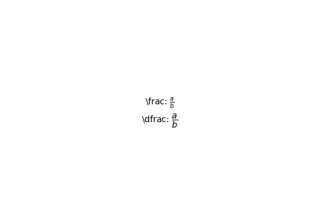The difference between \dfrac and \frac¶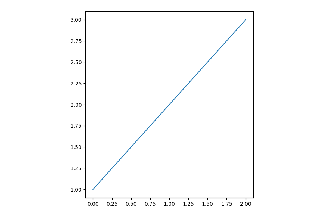Axes with a fixed physical size¶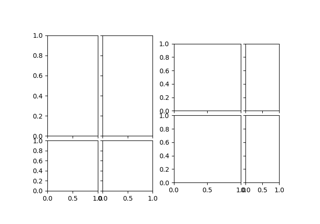Setting a fixed aspect on ImageGrid cells¶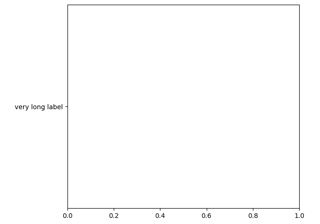Make Room For Ylabel Using Axesgrid¶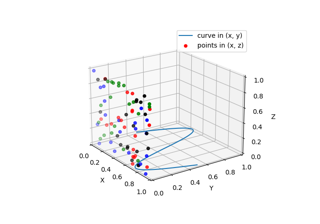Plot 2D data on 3D plot¶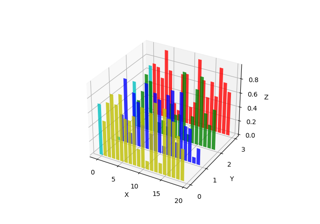Create 2D bar graphs in different planes¶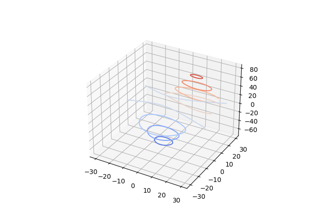Demonstrates plotting contour (level) curves in 3D¶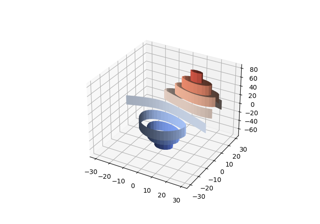Demonstrates plotting contour (level) curves in 3D using the extend3d option¶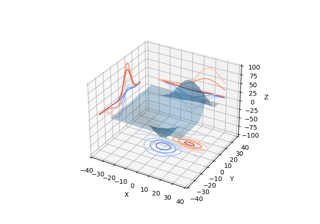Projecting contour profiles onto a graph¶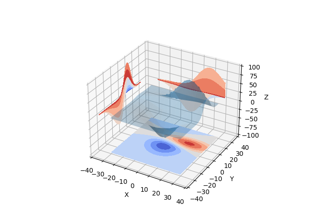Projecting filled contour onto a graph¶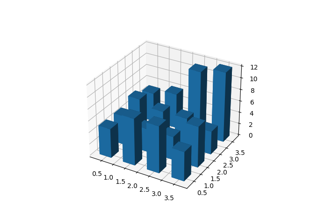Create 3D histogram of 2D data¶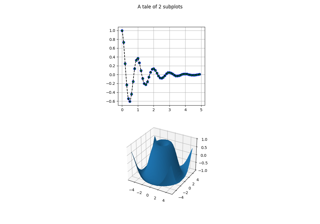2D and 3D Axes in same Figure¶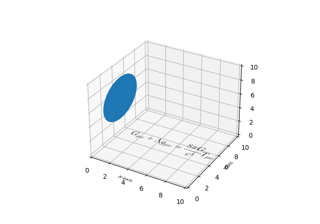Draw flat objects in 3D plot¶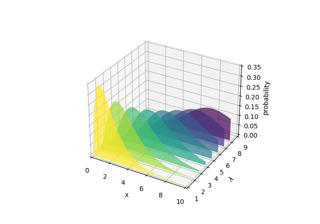Generate polygons to fill under 3D line graph¶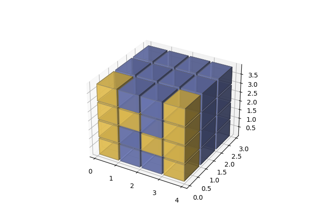3D voxel plot of the numpy logo¶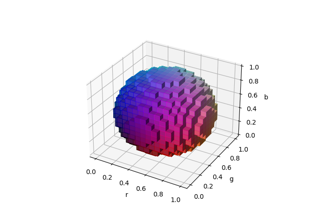3D voxel / volumetric plot with rgb colors¶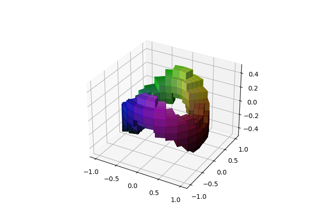3D voxel / volumetric plot with cylindrical coordinates¶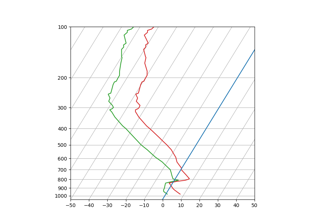SkewT-logP diagram: using transforms and custom projections¶